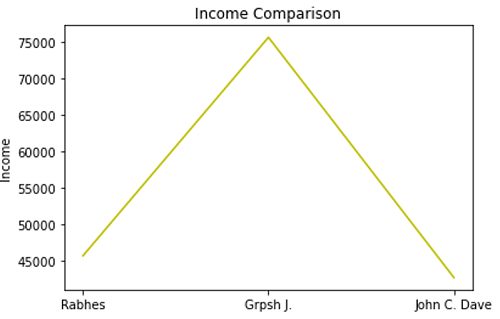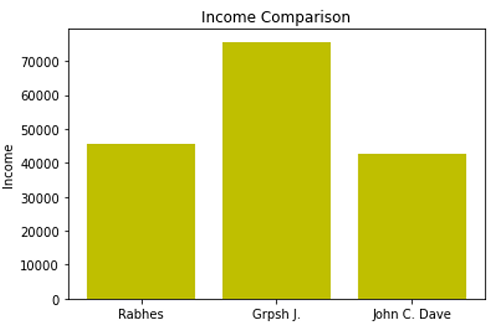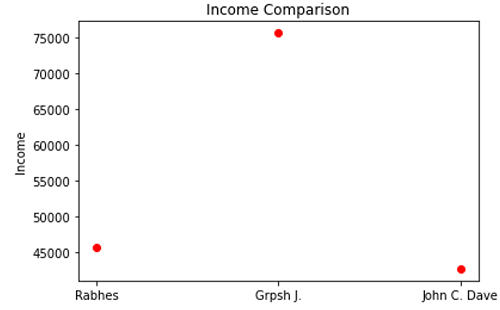# Python | Categorical Plotting

Python | Categorical Plotting: In this article, we are going to learn about the categorical plotting and its Python implementation.
Submitted by Anuj Singh, on July 11, 2020

Visualizing different variables is also a part of basic plotting. Such variables can have different classes, for example, numerical or a category. Matplotlib has an important feature of Categorical Plotting. We can plot multiple categorical variables within different types of plots such as line, dot, bar, scatter, etc.

Following are some examples of categorical plotting:

Syntax:

```matplotlib.pyplot.figure(figsize=(4,3))

#figsize(float, float)
width, height in inches.
```

## Example 1: Line Plot## Example 2: Bar Plot## Example 3: Dot Plot## Python code for categorical plotting

```# Data Visualization using Python
# Categorical Plotting

import matplotlib.pyplot as plt

names = ['Rabhes', 'Grpsh J.', 'John C. Dave']
values = [45646, 75640, 42645]

# example 1
plt.figure()
plt.plot(names, values, color='y')
plt.ylabel('Income')
plt.title('Income Comparison')
plt.show()

# example 2
plt.figure()
plt.bar(names, values, color='y')
plt.ylabel('Income')
plt.title('Income Comparison')
plt.show()

# example 3
plt.figure()
plt.plot(names, values, 'or')
plt.ylabel('Income')
plt.title('Income Comparison')
plt.show()
```

Output:

```Output is as figure
```

Languages: » C » C++ » C++ STL » Java » Data Structure » C#.Net » Android » Kotlin » SQL
Web Technologies: » PHP » Python » JavaScript » CSS » Ajax » Node.js » Web programming/HTML
Solved programs: » C » C++ » DS » Java » C#
Aptitude que. & ans.: » C » C++ » Java » DBMS
Interview que. & ans.: » C » Embedded C » Java » SEO » HR
CS Subjects: » CS Basics » O.S. » Networks » DBMS » Embedded Systems » Cloud Computing
» Machine learning » CS Organizations » Linux » DOS
More: » Articles » Puzzles » News/Updates